# How to work with file handling in C++

• Difficulty Level : Hard
• Last Updated : 15 Jul, 2021

Prerequisite: File Handling through C++ Classes

In C++, files are mainly dealt by using three classes fstream, ifstream, ofstream available in fstream header file. In this post we will discuss how to store data using file handling.

Attention reader! Don’t stop learning now. Get hold of all the important DSA concepts with the DSA Self Paced Course at a student-friendly price and become industry ready.  To complete your preparation from learning a language to DS Algo and many more,  please refer Complete Interview Preparation Course.

In case you wish to attend live classes with experts, please refer DSA Live Classes for Working Professionals and Competitive Programming Live for Students.

The idea is to take an example of Book Database and implement it the same in C++. Below are the functionality used:

• How to Insert book record
• How to view all book record
• How to search book record
• How to delete book record
• How to update book record

Below is the implementation of file handling operations:

## C++

 `// C++ program for implementing the above` `// mentioned functionalities` `#include ` `#include ` `#include ` `using` `namespace` `std;`   `// Book class` `class` `Book {` `private``:` `    ``int` `bookid;`   `    ``// Max book title size 20` `    ``char` `title;` `    ``float` `price;`   `public``:` `    ``// Default Constructor` `    ``Book()` `    ``{` `        ``bookid = 0;` `        ``strcpy``(title, ``"no title"``);` `        ``price = 0;` `    ``}`   `    ``// Function for taking book data` `    ``void` `getBookData()` `    ``{` `        ``cout << ``"Enter bookid, title, "` `             ``<< ``" price: "``;` `        ``cin >> bookid;` `        ``cin.ignore();` `        ``cin.getline(title, 19);` `        ``cin >> price;` `    ``}`   `    ``// Function for showing book data` `    ``void` `showBookData()` `    ``{` `        ``cout << ``"\n"` `             ``<< bookid` `             ``<< ``" "` `<< title << ``" "` `<< price;` `    ``}`   `    ``int` `storeBook();` `    ``void` `viewAllBooks();` `    ``void` `searchBook(``char``*);` `    ``void` `deleteBook(``char``*);` `    ``void` `updateBook(``char``*);` `};`   `// Function for update book data` `void` `Book::updateBook(``char``* t)` `{` `    ``fstream file;`   `    ``// Open the file` `    ``file.open(``"myfile3.txt"``,` `              ``ios::in | ios::out | ios::ate | ios::binary);` `    ``file.seekg(0);` `    ``file.read((``char``*)``this``, ``sizeof``(*``this``));`   `    ``// Read the file` `    ``while` `(!file.eof()) {` `        ``if` `(!``strcmp``(t, title)) {` `            ``getBookData();`   `            ``file.seekp(file.tellp() - ``sizeof``(*``this``));` `            ``file.write((``char``*)``this``, ``sizeof``(*``this``));` `        ``}` `        ``file.read((``char``*)``this``, ``sizeof``(*``this``));` `    ``}`   `    ``// Close the file` `    ``file.close();` `}`   `// Function for delete book data` `void` `Book::deleteBook(``char``* t)` `{` `    ``ifstream fin;` `    ``ofstream fout;` `    ``fin.open(``"myfile3.txt"``,` `             ``ios::app | ios::binary);` `    ``if` `(!fin)` `        ``cout << ``"\n File not found"``;` `    ``else` `{` `        ``fout.open(``"tempfile.txt"``,` `                  ``ios::app | ios::binary);` `        ``fin.read((``char``*)``this``, ``sizeof``(*``this``));`   `        ``// Until end of file is not reached` `        ``while` `(!fin.eof()) {` `            ``if` `(``strcmp``(title, t))` `                ``fout.write((``char``*)``this``, ``sizeof``(*``this``));` `            ``fin.read((``char``*)``this``, ``sizeof``(*``this``));` `        ``}` `        ``fin.close();` `        ``fout.close();` `        ``remove``(``"myfile3.txt"``);` `        ``rename``(``"tempfile.txt"``, ``"myfile3.txt"``);` `    ``}` `}`   `// Function to search the Book` `void` `Book::searchBook(``char``* t)` `{` `    ``int` `counter = 0;` `    ``ifstream fin;` `    ``fin.open(``"myfile3.txt"``,` `             ``ios::in | ios::binary);`   `    ``// If file is not found` `    ``if` `(!fin)` `        ``cout << ``"File not found"``;` `    ``else` `{` `        ``fin.read((``char``*)``this``, ``sizeof``(*``this``));`   `        ``// Until end of file is not reached` `        ``while` `(!fin.eof()) {` `            ``if` `(!``strcmp``(t, title)) {` `                ``showBookData();` `                ``counter++;` `            ``}` `            ``fin.read((``char``*)``this``, ``sizeof``(*``this``));` `        ``}` `        ``if` `(counter == 0)` `            ``cout << ``"No record found"``;` `        ``fin.close();` `    ``}` `}`   `// Function to view all the Book` `void` `Book::viewAllBooks()` `{` `    ``ifstream fin;`   `    ``// Open function open file named` `    ``// myfile.txt` `    ``fin.open(``"myfile3.txt"``, ios::in | ios::binary);` `    ``if` `(!fin)` `        ``cout << ``"File not found"``;`   `    ``else` `{` `        ``fin.read((``char``*)``this``, ``sizeof``(*``this``));`   `        ``// Until end of file is` `        ``// not reached` `        ``while` `(!fin.eof()) {` `            ``showBookData();`   `            ``// read is object of ifstream` `            ``// class which is used for` `            ``// read data of file` `            ``fin.read((``char``*)``this``, ``sizeof``(*``this``));` `        ``}` `        ``fin.close();` `    ``}` `}`   `// Function to implement the store of` `// all the books` `int` `Book::storeBook()` `{` `    ``if` `(bookid == 0 && price == 0) {` `        ``cout << ``"book data not"` `             ``<< ``" initialized"``;` `        ``return` `(0);` `    ``}` `    ``else` `{` `        ``ofstream fout;`   `        ``// In the below line open function` `        ``// used to open files. If the file` `        ``// does not exist then it will` `        ``// create the file "myfile.txt"` `        ``fout.open(``"myfile3.txt"``, ios::app | ios::binary);`   `        ``// Write function is used for` `        ``// data to write in the file` `        ``fout.write((``char``*)``this``, ``sizeof``(*``this``));` `        ``fout.close();` `        ``return` `(1);` `    ``}` `}`   `// Function to display the menu of the` `// current menu-driver` `int` `menu()` `{` `    ``int` `choice;` `    ``cout << ``"\nBook Management"``;` `    ``cout << ``"\n1.Insert book record"``;` `    ``cout << ``"\n2.View all book record"``;` `    ``cout << ``"\n3.Search book record"``;` `    ``cout << ``"\n4.Delete book record"``;` `    ``cout << ``"\n5.Update book record"``;` `    ``cout << ``"\n6.Exit"``;` `    ``cout << ``"\n\nEnter your choice : "``;` `    ``cin >> (choice);` `    ``return` `(choice);` `}`   `// Driver Code` `int` `main()` `{`   `    ``// Object of the class Book` `    ``Book b1;` `    ``char` `title;`   `    ``while` `(1) {` `        ``system``(``"cls"``);` `        ``switch` `(menu()) {` `        ``case` `1:` `            ``b1.getBookData();` `            ``b1.storeBook();` `            ``cout << ``"\nRecord inserted"``;` `            ``break``;`   `        ``case` `2:` `            ``b1.viewAllBooks();` `            ``break``;`   `        ``case` `3:` `            ``cout << ``"\nEnter title of "` `                 ``<< ``"book to search : "``;` `            ``cin.ignore();` `            ``cin.getline(title, 19);` `            ``b1.searchBook(title);` `            ``break``;`   `        ``case` `4:` `            ``cout << ``"\nEnter book title "` `                 ``<< ``"for delete record : "``;` `            ``cin.ignore();` `            ``cin.getline(title, 19);` `            ``b1.deleteBook(title);` `            ``break``;`   `        ``case` `5:` `            ``cout << ``"\nEnter book title "` `                 ``<< ``"to update record : "``;` `            ``cin.ignore();` `            ``cin.getline(title, 19);` `            ``b1.updateBook(title);` `            ``break``;`   `        ``case` `6:` `            ``cout << ``"\n Thanks for using"``;` `            ``cout << ``"\n Press any key to exit"``;` `            ``getch();` `            ``exit``(0);`   `        ``default``:` `            ``cout << ``"Invalid input"``;` `        ``}` `        ``getch();` `    ``}` `}`

Output:

• Output for inserting book record using choice 1: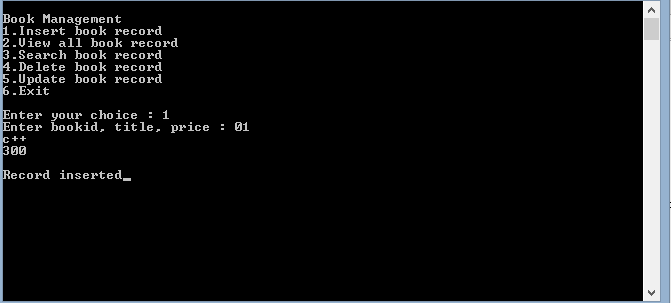• Output after again inserting book record using choice 1: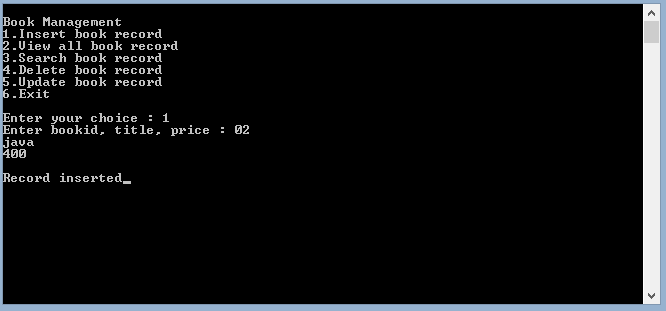• Output for inserting record 3, 4 and we can show record using the choice 2. View all book record. If “0” is appended as prefix of bookid then it is ignored as we can see in below image: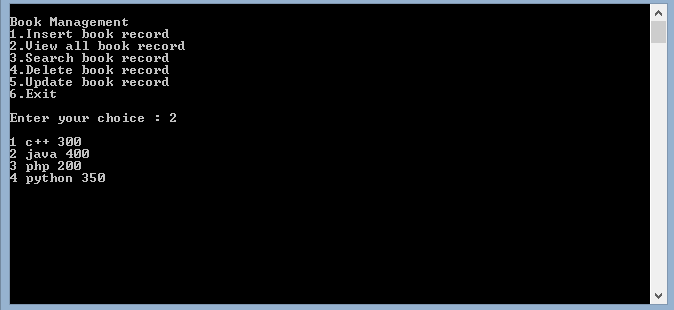• Output for the searching book using its title name using choice 3: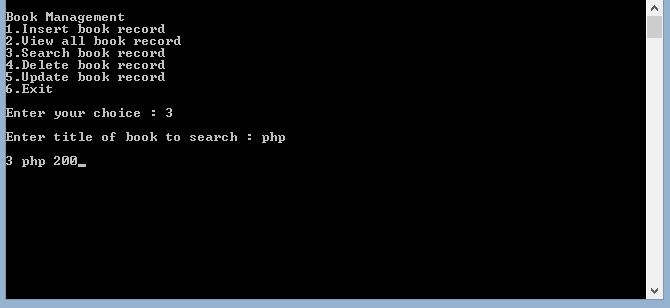• Output for updating the previously inserted data as shown below: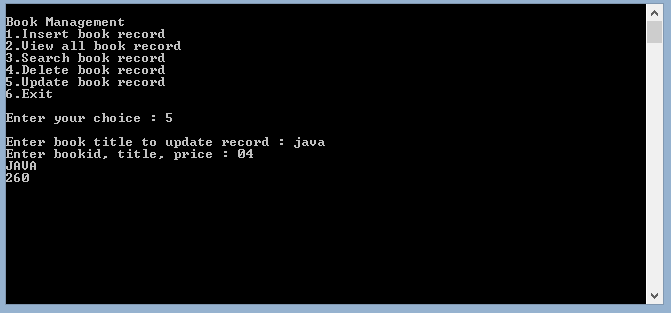• Now finally it can be seen in below image “java” is updated as “JAVA” as well as book id as 4 and price as “350”. The previous data of java book in 3rd image can be seen. The record for C++ is deleted that is why it is not shown below: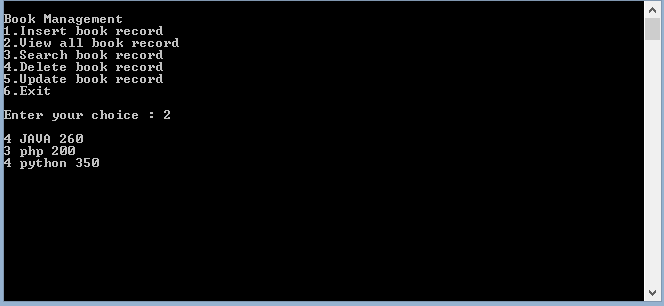My Personal Notes arrow_drop_up
Recommended Articles
Page :